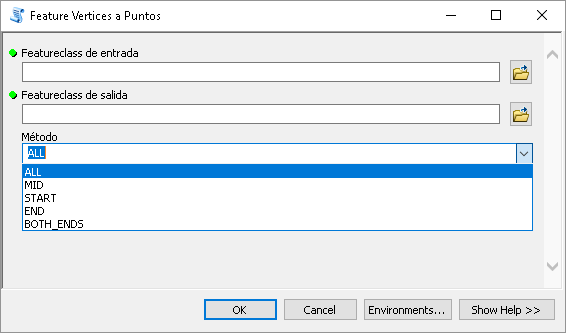# Want FeatureVerticesToPoints_management functionality in ArcGIS 10.1 Standard or any other alternative to get points of vertices

1793
9
11-09-2017 02:41 AMNew Contributor II

Hello Everyone,

I need to run arcpy.FeatureVerticesToPoints_management() method in ArcGIS 10.1 Desktop Standard, but it has License just for ArcGIS Desktop Advanced. Can anyone suggest me any other alternative to get the point layer of the vertices like I get in  FeatureVerticesToPoints_management() method..

Thanks and Regards..

1 Solution

Accepted SolutionsbyEsri Esteemed Contributor

Find below the code that I used to recreate the Feature Vertices To Points tool for Basic licenses (sorry for the Spanish interface):Code for the tool:

``````#-------------------------------------------------------------------------------
# Name:        FeatureVerticesToPoints.py
# Purpose:     Convertir los vertices de features a puntos
#
# Author:      xbakker
#
# Created:     27/02/2015
#-------------------------------------------------------------------------------
import arcpy
import os

def main():

# parameters
fc_in = arcpy.GetParameterAsText(0)
fc_out = arcpy.GetParameterAsText(1)
method = arcpy.GetParameterAsText(2)

# fld para poder hacer el join con la entrada

# geometria de salida
geomtype = "POINT"
sr = arcpy.Describe(fc_in).spatialReference

# recreate fc_out based on fc_lines (in)
ws_name, fc_name = os.path.split(fc_out)
createFeatureClass(fc_out, fc_in, geomtype, sr)

# cursors:
cnt = 0
flds_out = ("SHAPE@", fld_oid_in)
with arcpy.da.InsertCursor(fc_out, flds_out) as curs_out:
flds_in =("SHAPE@", "OID@")
with arcpy.da.SearchCursor(fc_in, flds_in) as curs:
for row in curs:
feat = row
oid = row

# get vertices
lst_vertices = getVertices(feat, method)

# store vertices
for vertice in lst_vertices:
cnt += 1
if cnt % 100 == 0:
curs_out.insertRow((vertice, oid, ))

def getVertices(feat, method):
"""Convertir poligono o linea a puntos dependiendo del metodo"""
if feat.type == "polygon":
polyline = feat.boundary()
else:
polyline = feat

lst_vertices = []
if method == "ALL":
for part in polyline:
for pnt in part:
lst_vertices.append(pnt)

elif method == "MID":
pnt = polyline.positionAlongLine(0.5, True)
lst_vertices.append(pnt)

elif method == "START":
lst_vertices.append(polyline.firstPoint)

elif method == "END":
lst_vertices.append(polyline.lastPoint)

elif method == "BOTH_ENDS":
lst_vertices.append(polyline.firstPoint)
lst_vertices.append(polyline.lastPoint)

return lst_vertices

def createFeatureClass(fc_out, fc_in, geomtype, sr):
ws_name, fc_name = os.path.split(fc_out)
arcpy.CreateFeatureclass_management(ws_name, fc_name, geomtype, spatial_reference=sr)

if __name__ == '__main__':
main()‍‍‍‍‍‍‍‍‍‍‍‍‍‍‍‍‍‍‍‍‍‍‍‍‍‍‍‍‍‍‍‍‍‍‍‍‍‍‍‍‍‍‍‍‍‍‍‍‍‍‍‍‍‍‍‍‍‍‍‍‍‍‍‍‍‍‍‍‍‍‍‍‍‍‍‍‍‍‍‍‍‍‍‍‍‍‍‍‍‍``````
9 RepliesbyMVP Legendary Contributor

FeatureClassToNumPyArray with the explode_to_points option set to True

http://desktop.arcgis.com/en/arcmap/latest/analyze/arcpy-data-access/featureclasstonumpyarray.htm

Then Numpyarraytofeatureclass to get the exploded geometry back to points.

Or some searchcursor approach doing effectively what the above doesNew Contributor II

Sir,As per your approach,The coordinates I am getting are of the center of the lines, Is there any way to get the vertices of the line i.e. the start and the end points of the line.by
New Contributor III

You can create two fields for start point and two fields for end points of line (XStart / YStart / XEnd / YEnd), and use 'Calculate Geometry' to get this coordinates.byMVP Legendary Contributor

You didn't specify the explode_to_points=True (check http://desktop.arcgis.com/en/arcmap/latest/analyze/arcpy-data-access/featureclasstonumpyarray.htm

that is why you are getting the centroid onlyby
New Contributor III

If you need only the Coordinates of points and working with Enterprise Geodatabase, you can read the coordinates with a Query layer or using the RDBMS tools with a function like ST_ASTEXT and getting all of the coordinates.byEsri Esteemed Contributor

Find below the code that I used to recreate the Feature Vertices To Points tool for Basic licenses (sorry for the Spanish interface):Code for the tool:

``````#-------------------------------------------------------------------------------
# Name:        FeatureVerticesToPoints.py
# Purpose:     Convertir los vertices de features a puntos
#
# Author:      xbakker
#
# Created:     27/02/2015
#-------------------------------------------------------------------------------
import arcpy
import os

def main():

# parameters
fc_in = arcpy.GetParameterAsText(0)
fc_out = arcpy.GetParameterAsText(1)
method = arcpy.GetParameterAsText(2)

# fld para poder hacer el join con la entrada

# geometria de salida
geomtype = "POINT"
sr = arcpy.Describe(fc_in).spatialReference

# recreate fc_out based on fc_lines (in)
ws_name, fc_name = os.path.split(fc_out)
createFeatureClass(fc_out, fc_in, geomtype, sr)

# cursors:
cnt = 0
flds_out = ("SHAPE@", fld_oid_in)
with arcpy.da.InsertCursor(fc_out, flds_out) as curs_out:
flds_in =("SHAPE@", "OID@")
with arcpy.da.SearchCursor(fc_in, flds_in) as curs:
for row in curs:
feat = row
oid = row

# get vertices
lst_vertices = getVertices(feat, method)

# store vertices
for vertice in lst_vertices:
cnt += 1
if cnt % 100 == 0:
curs_out.insertRow((vertice, oid, ))

def getVertices(feat, method):
"""Convertir poligono o linea a puntos dependiendo del metodo"""
if feat.type == "polygon":
polyline = feat.boundary()
else:
polyline = feat

lst_vertices = []
if method == "ALL":
for part in polyline:
for pnt in part:
lst_vertices.append(pnt)

elif method == "MID":
pnt = polyline.positionAlongLine(0.5, True)
lst_vertices.append(pnt)

elif method == "START":
lst_vertices.append(polyline.firstPoint)

elif method == "END":
lst_vertices.append(polyline.lastPoint)

elif method == "BOTH_ENDS":
lst_vertices.append(polyline.firstPoint)
lst_vertices.append(polyline.lastPoint)

return lst_vertices

def createFeatureClass(fc_out, fc_in, geomtype, sr):
ws_name, fc_name = os.path.split(fc_out)
arcpy.CreateFeatureclass_management(ws_name, fc_name, geomtype, spatial_reference=sr)

if __name__ == '__main__':
main()‍‍‍‍‍‍‍‍‍‍‍‍‍‍‍‍‍‍‍‍‍‍‍‍‍‍‍‍‍‍‍‍‍‍‍‍‍‍‍‍‍‍‍‍‍‍‍‍‍‍‍‍‍‍‍‍‍‍‍‍‍‍‍‍‍‍‍‍‍‍‍‍‍‍‍‍‍‍‍‍‍‍‍‍‍‍‍‍‍‍``````New Contributor II

Thank you very much sir, My problem is solved now.by
New Contributor II

hey, i cannot run that script it wont run .

here is the error:any help would be appriciate!

thanks!New Contributor II

I'm getting the same error with this code. It worked for me in ArcMap but not when I run it in Pro.

Could it be differences between Python 2 and 3? I'm not familiar enough with syntax differences between the two that might be tripping me up in Pro. Any ideas?